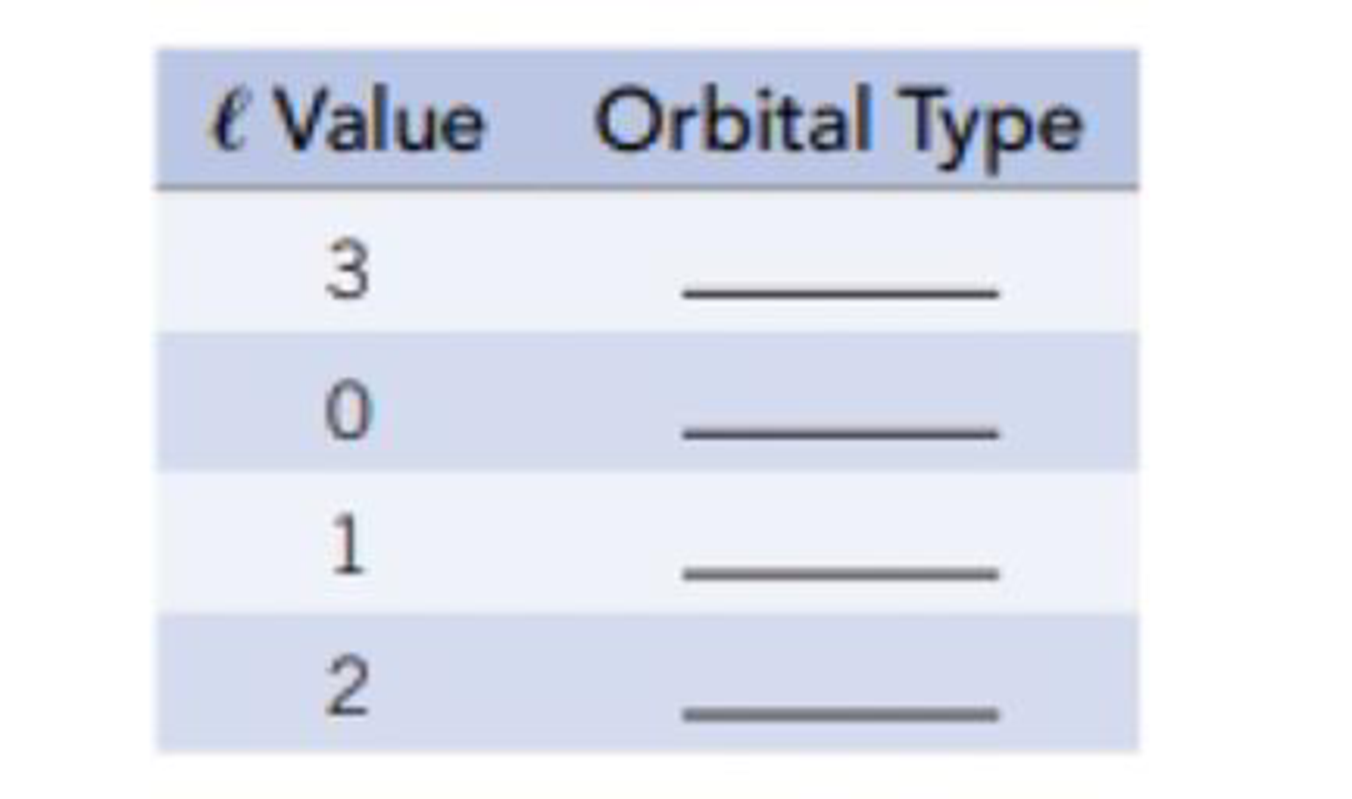# Match the values of l shown in the table with orbital type ( s , p , d , or f ).### Chemistry & Chemical Reactivity

9th Edition
John C. Kotz + 3 others
Publisher: Cengage Learning
ISBN: 9781133949640

#### Solutions

Chapter
Section### Chemistry & Chemical Reactivity

9th Edition
John C. Kotz + 3 others
Publisher: Cengage Learning
ISBN: 9781133949640
Chapter 6, Problem 51GQ
Textbook Problem
13 views

## Match the values of l shown in the table with orbital type (s, p, d, or f).Interpretation Introduction

Interpretation: The orbitals for the given values of l should be identified.

Concept introduction:

Quantum numbers are numbers, which explains the existence and the behavior of electron in an atom.

1. a) Principle quantum number is represented by n and this number describes the energy of the orbital and the size of an atom.
2. b) Angular momentum quantum number (or azimuthal quantum number) is represented by l and this number indicates the shape of the orbitals.
3. c) Magnetic quantum number is represented by ml and this number indicates the orientation of the orbital.
4. d) Spin quantum number is represented by ms and this number indicates the spin of the electron.

Angular momentum quantum number (or azimuthal quantum number) is represented by l and this number indicates the shape of the orbitals. Each value of l indicates subshell.

### Explanation of Solution

Angular momentum quantum number (or azimuthal quantum number) is represented by l and this number indicates the shape of the orbitals.

The values of l when the principal quantum number is n are from 0 to (n1). Each value of l indicates subshell.

Therefore for l=0,1,2 and 3 represents s, p, d and f subshells For p subshells, l=1

### Still sussing out bartleby?

Check out a sample textbook solution.

See a sample solution

#### The Solution to Your Study Problems

Bartleby provides explanations to thousands of textbook problems written by our experts, many with advanced degrees!

Get Started

Find more solutions based on key concepts
According to genomic researchers, a single inherited gene is the probable cause of common obesity. T F

Nutrition: Concepts and Controversies - Standalone book (MindTap Course List)

In which domain and kingdom are humans classified?

Biology: The Dynamic Science (MindTap Course List)

2-25 Name and give the symbol for each element in Problem 2-24.

Introduction to General, Organic and Biochemistry

What is the difference between type I and type II supernovae?

Horizons: Exploring the Universe (MindTap Course List)

Tell how you would try to prove to a doubter that air is matter.

Chemistry for Today: General, Organic, and Biochemistry

A square hole 8.00 cm along each side is cut in a sheet of copper. (a) Calculate the change in the area of this...

Physics for Scientists and Engineers, Technology Update (No access codes included)

How does metabolic rate vary with temperature?

Oceanography: An Invitation To Marine Science, Loose-leaf Versin

Bacteria, Archaea, and Eukarya are three ________.

Biology: The Unity and Diversity of Life (MindTap Course List)

Four properties of gases may be measured. Name them.

Introductory Chemistry: An Active Learning Approach